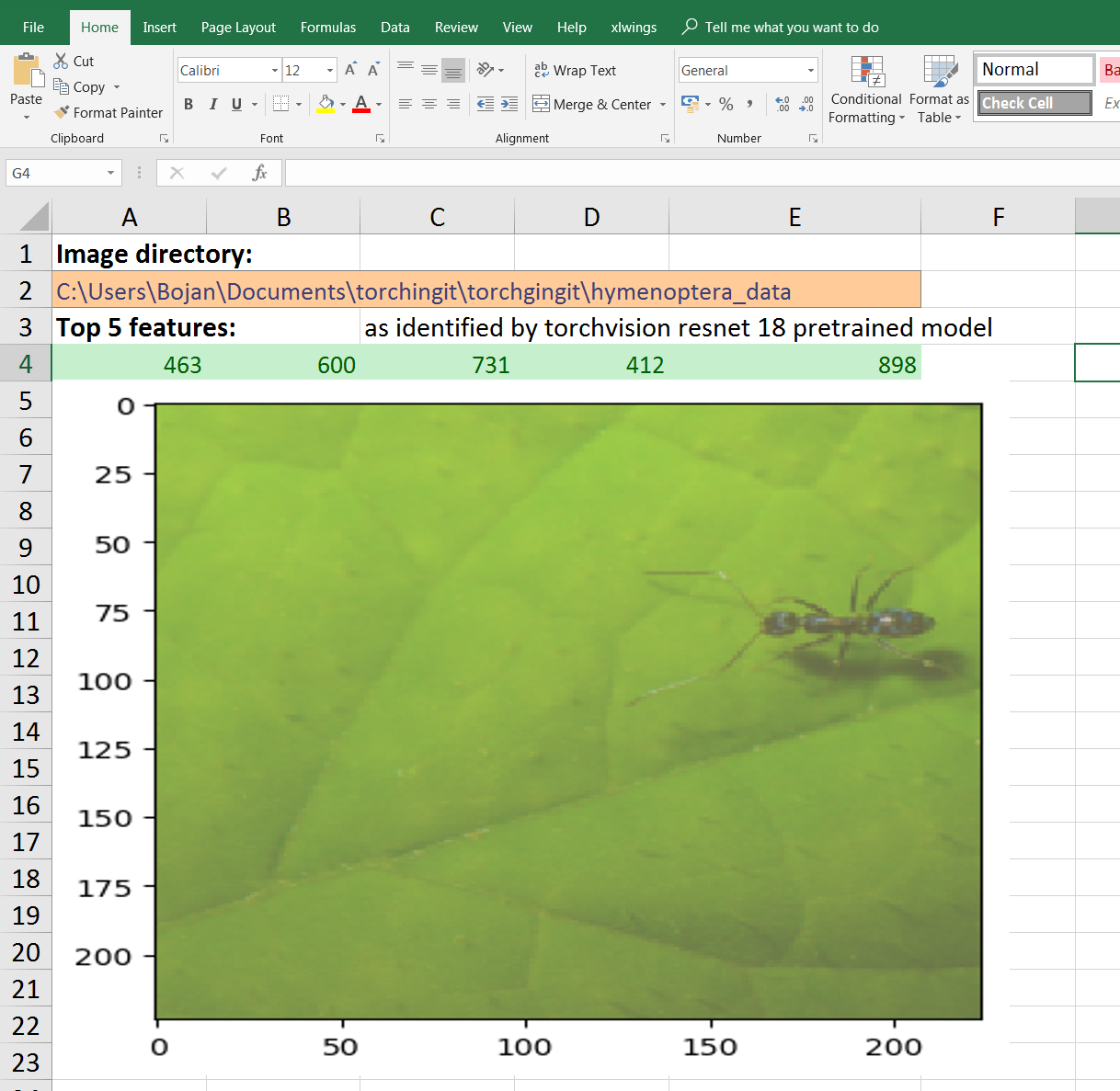# Using PyTorch from Microsoft Excel

Using Python from within Excel has long been attractive, in particular in order to leverage the large number of Python packages for numerical and other data processing. I made my own small contribution to this a while ago with the (ExPy)[http://www.bnikolic.co.uk/expy/expy.html] project. In recent time the independently made xlwings has become my main tool for this and now works very well indeed (certainly I’d recommend it over ExPy!).

On the Python side I’ve been increasing been using PyTorch not just for machine learning but to accelerate general numerical processing (see for example this paper ). Not surprisingly, the two can be effectively be married to cutting-edge neural network machine learning directly from Excel! Here is how.

## Setup

1. First and most obvious is installing Excel. PyTorch for Microsoft Windows is distributed as a 64bit program only, so 64bit version Excel is required for in-processes use. Note that the default version of Office is 32bit, so if you have this version you will need to reinstall with the 64bit version.
2. Second task is install Python. I installed the official Python for Windows from https://www.python.org/downloads/windows/ . I installed the latest Python V3.7.0
3. Next job is to install PyTorch and other Python modules – this is extremely easy using the built in pip3 tool:
``````pip3 install pytorch-cpu, torchvision,  xlwings, numpy, mathplotlib
``````

## Example Application

For a simple example spreadsheet I’ve adapted some code from this tuorial . It shows use of a pre-trained neural network to extract features from some ImageNet images.

Here is a screen grab of the Excel:Some of the features it shows:

• Use of named cells to do configuration (e.g., location of the directory containing the image data)

• Matplotlib plotting with the figures inserted straight into Excel

• Use of Python based UDFs for clean, functional interface

• Exchange of data as numpy arrays / Excel array formulae

Here is the Python code:

``````
# Bojan Nikolic <bojan@bnikolic.co.uk> 2018
#
# See https://pytorch.org/tutorials/beginner/transfer_learning_tutorial.html
# for script used as inspiration. Code from there used under BSD license

from __future__ import print_function, division

import os

import numpy
from matplotlib import pylab

import xlwings as xw

import torch
import torchvision
from torchvision.transforms import transforms as TF

_std = numpy.array([0.229, 0.224, 0.225])
_mean = numpy.array([0.485, 0.456, 0.406])

_m1 = torchvision.models.resnet18(pretrained=True)

dtrans=TF.Compose([
TF.Resize(256),
TF.CenterCrop(224),
TF.ToTensor(),
TF.Normalize(_std,
_mean)])

def imshow(t,
title=None):
t=t.numpy().transpose((1, 2, 0))
t=_std * t + _mean
t=numpy.clip(t, 0, 1)
pylab.imshow(t)
if title:
pylab.title(title)

dtrans)

batch_size=1,
shuffle=True,
num_workers=0)

def topf(t, m, N=5):
"""Top features of model m evaluated on t"""
r=torch.topk(_m1(t),
N)
return (r.detach().numpy(),
r.detach().numpy())

@xw.func
def tclass():